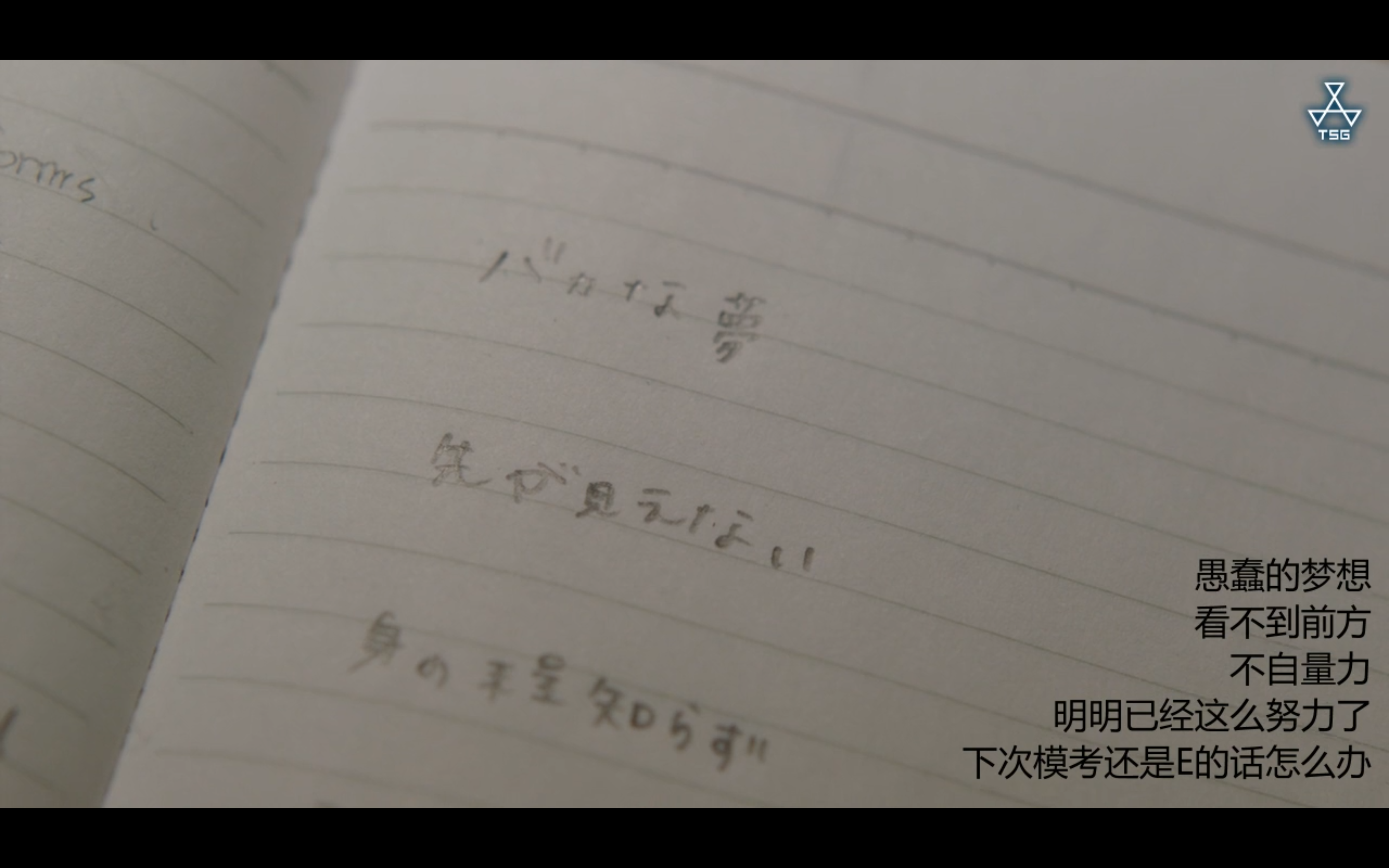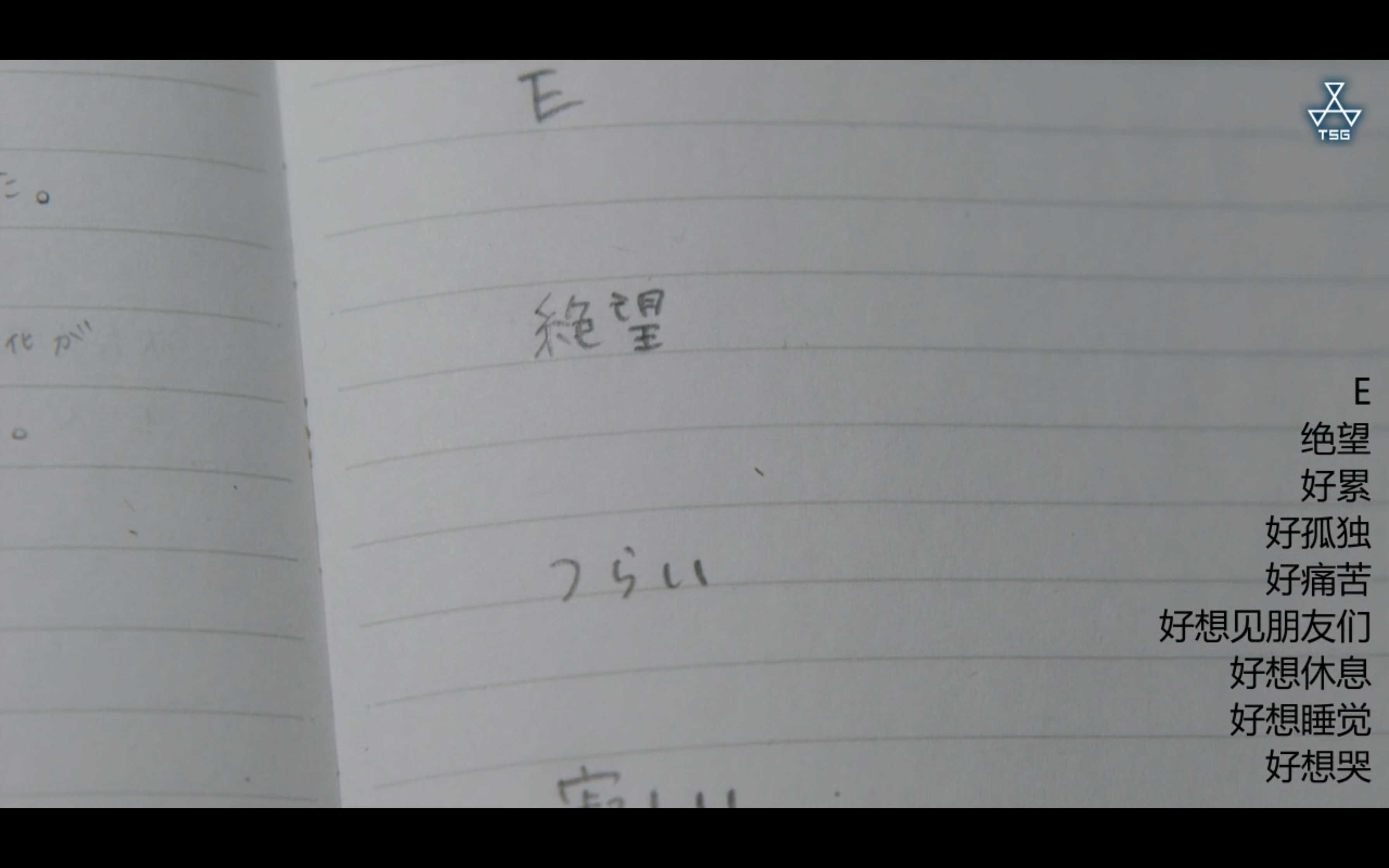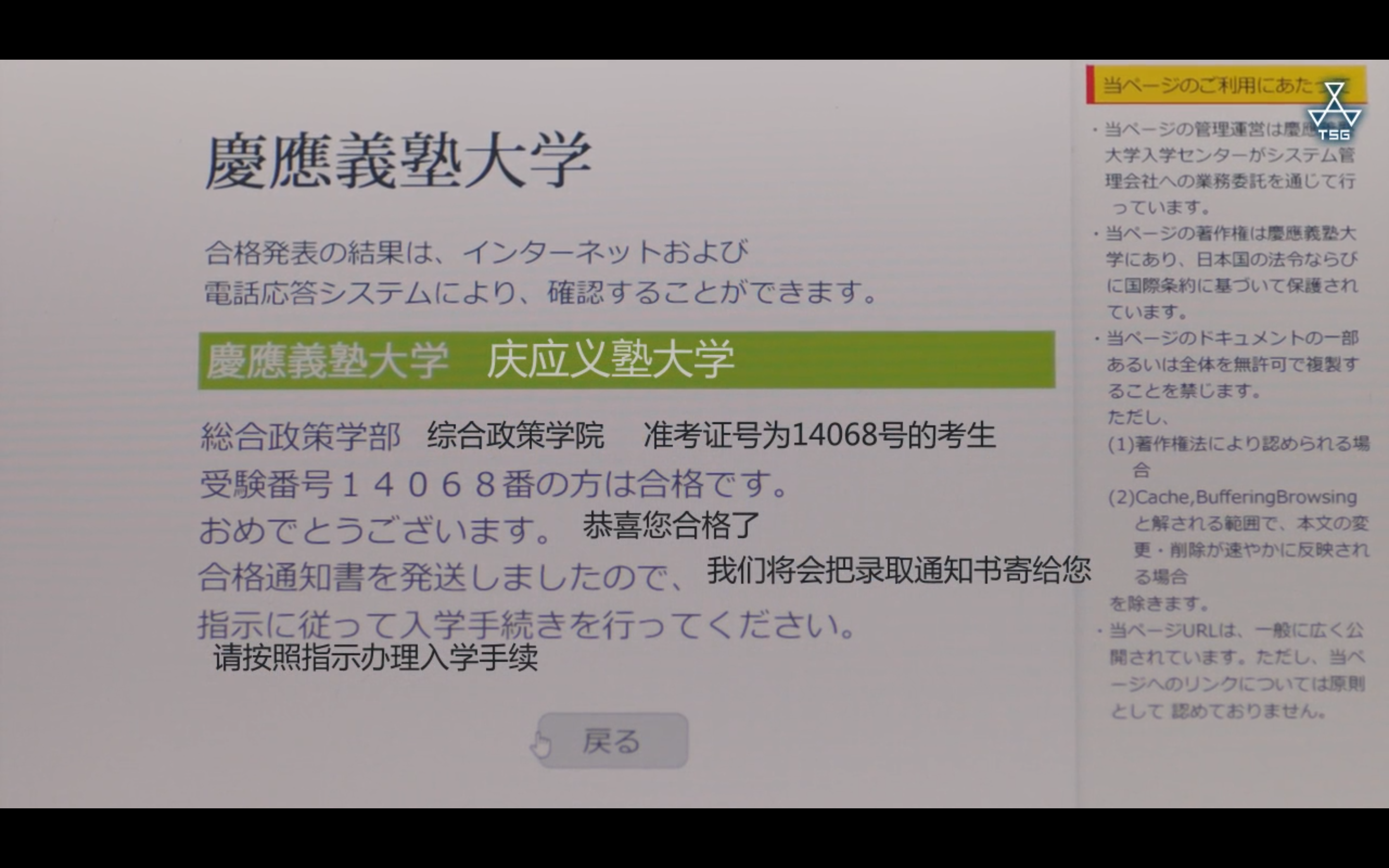# 关于正态分布表的一个有趣发现

· 阅读需 9 分钟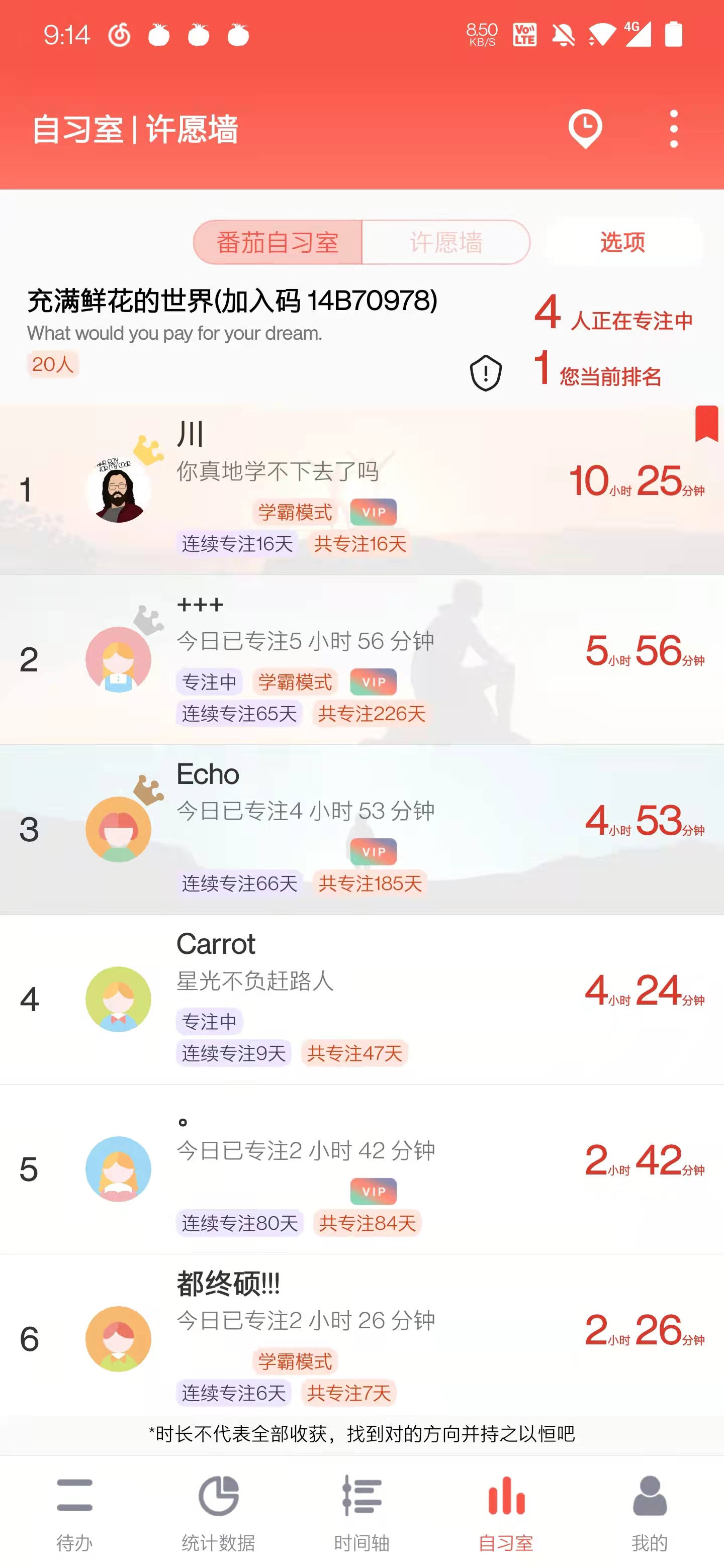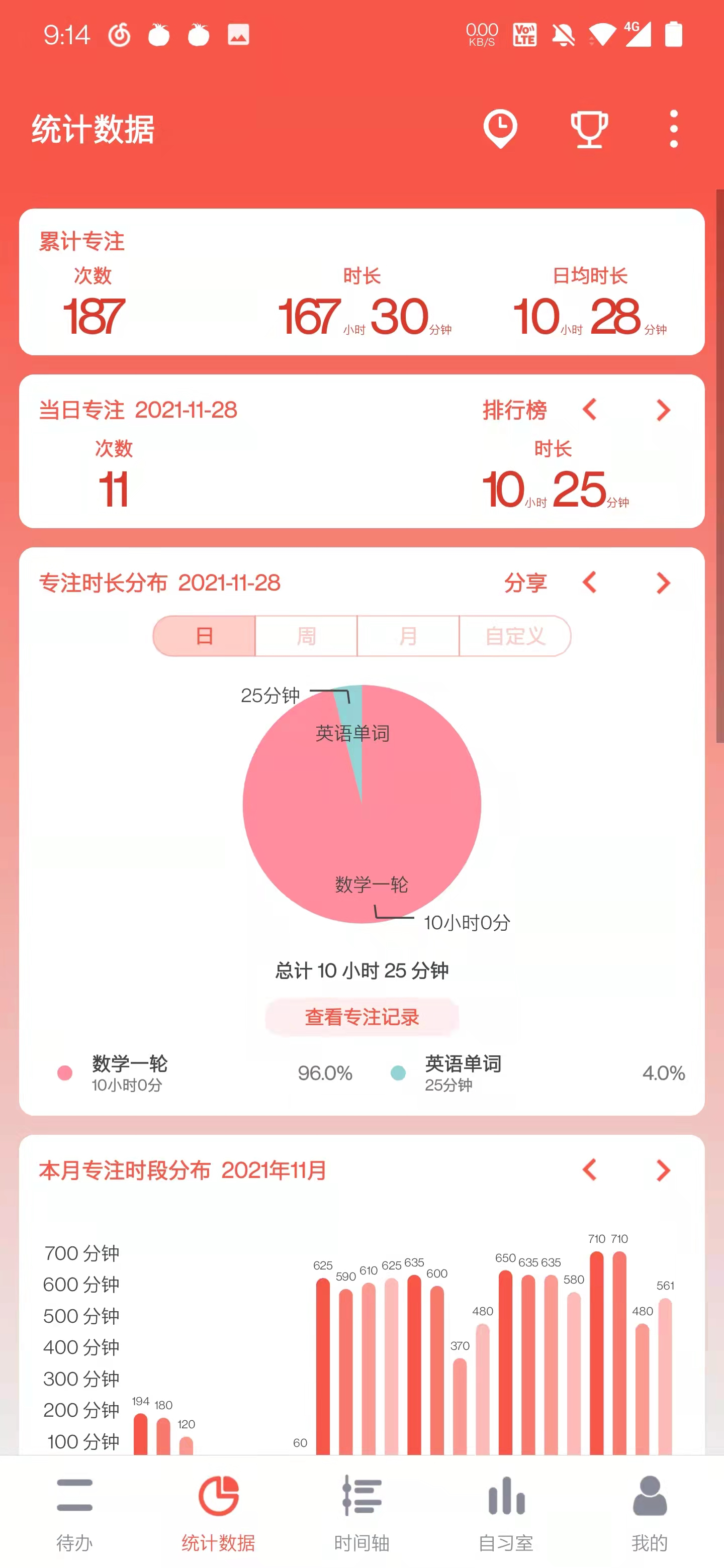## 关于正态分布表的一个有趣发现​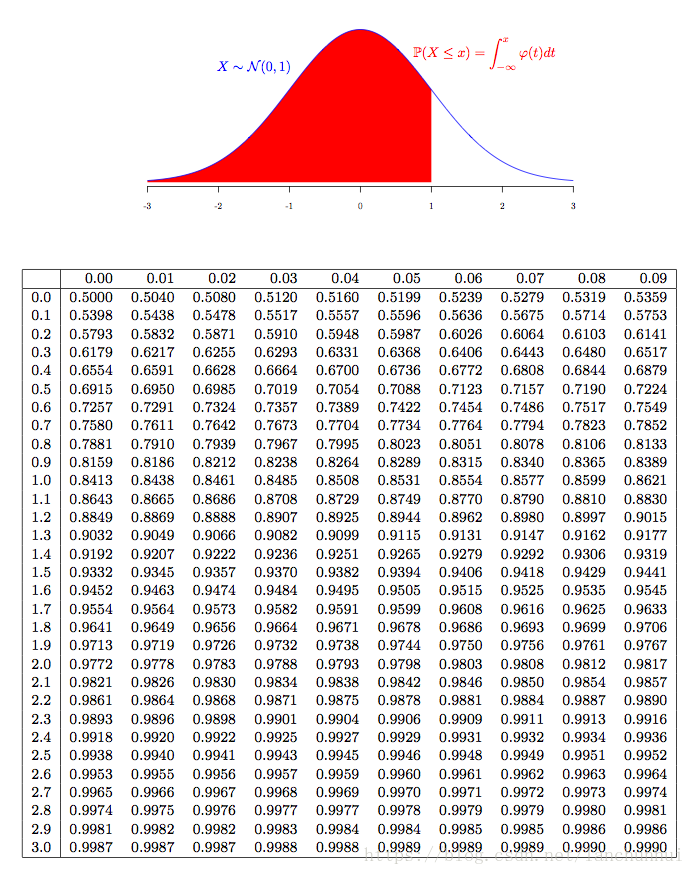$$n = 2 \times 4 = 8\ di = 0.1, 0.09, ..., \ \sum{i=1}^{n} {di}=\dfrac{(0.10+0.02)*9}{2} - 0.06 = 0.48\ \therefore \Phi(2) =\Phi(0)+\sum{i=1}^{n}{d_i}= 0.5 + 0.48 = 0.98$$

x\Phi(x,2)$$\Delta \Phi(x, 2) 00.5 0.250.6+0.1 0.50.69+0.09 0.750.77+0.08 10.84+0.07 1.250.89+0.05 1.50.93+0.04 1.750.96+0.03 20.98+0.02 2.250.99+0.01 2.50.99+0 2.751 31 ## 关于昨天那道题​ ### 题目​ 甲乙二人轮流投篮，甲先开始，甲每轮投一次，乙每轮投两次，甲乙每次投篮命中率分别为 p 和 0.5，求当 p 等于多少时，甲乙胜负概率相等 ### 解答​$$ \begin{align} \because \left{ \begin{array}{l} P(甲胜) & = p + (1-p)\times \frac{1}{4}\times P(甲胜) & = \dfrac{4p}{3+p} \ P(乙胜) & = (1-p)\times \frac{3}{4} + (1-p)\times \frac{1}{4}\times P(乙胜) & = \dfrac{3(1-p)}{3+p} \ \end{array} \right . \newline \begin{array}{l} \therefore P(甲胜) = P(乙胜) & = > 4p = 3(1-p) & = > p = \frac{3}{7} &&&&&& \end{array} \end{align}$### 评注​ 这题来自全书，答案是用模拟每次投篮做的，写的很繁，但是用我们昨天讨论的不变式去做就非常简单与快速。 不过要小心，这种解法只适合“无记忆性”的题目，即新的每一轮几乎与过去没啥关系，假如我们加个约束条件，比如假定 10 轮内甲乙胜负概率相等，那就只能用模拟法了，因为已经发生的 k 轮对接下来的 10-k 轮的讨论可能有影响。 ## 关于无记忆性的指数分布​ 指数分布是无记忆性的，即当$x>0$时，$P(X>s+t | X>s)=P(X>t)\$，它常用来描述一些电子元器件的使用寿命。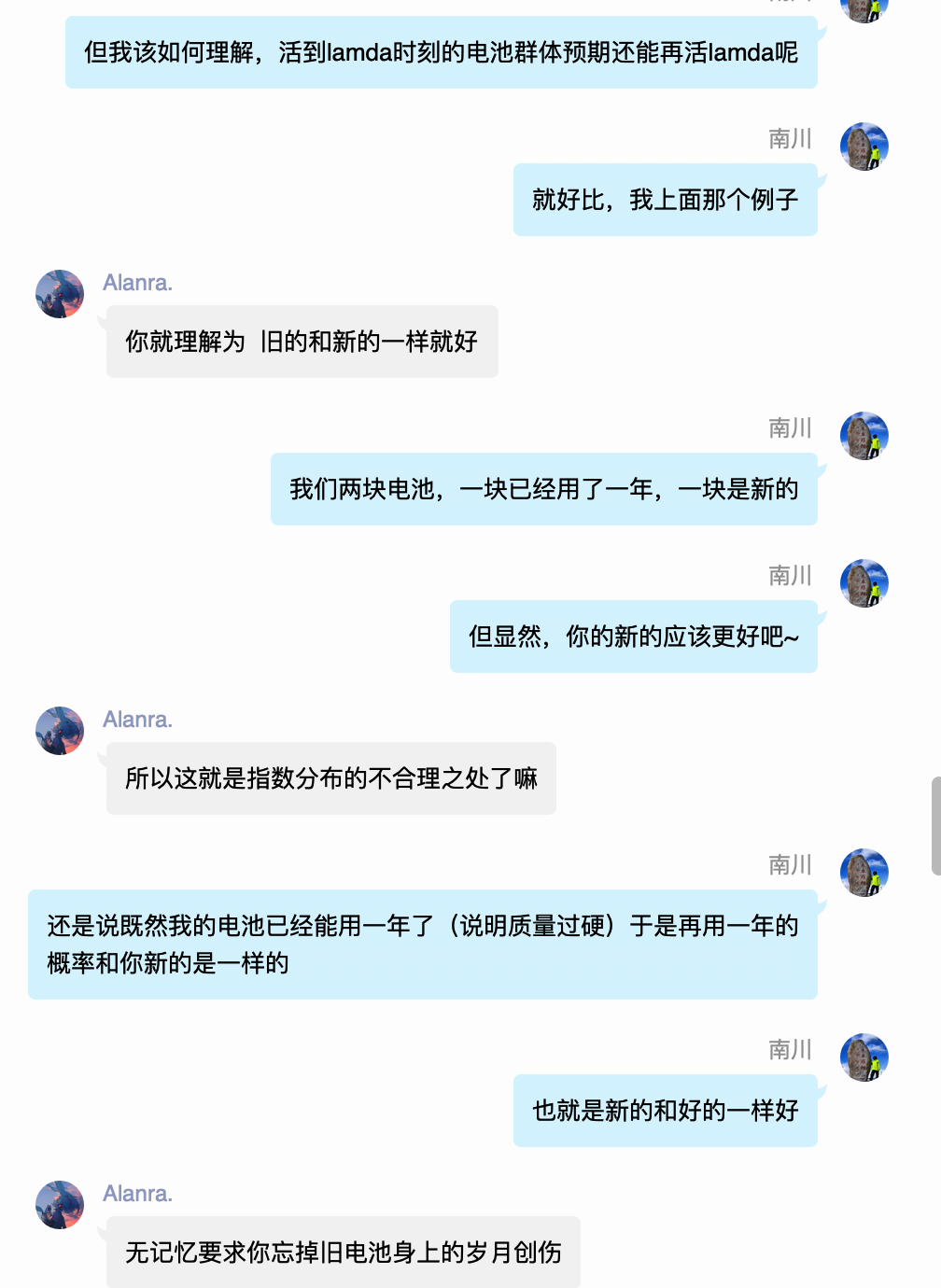## 最后，如果有一天你坚持不下去了……​